### FEA in One Dimension: One Dimensional Quadratic Elements

In the previous section, the domain of a one-dimensional problem was discretized using piecewise linear functions. Another approximation is the C0 piecewise quadratic interpolation functions, where on each element, the displacement is quadratic. If one wishes to obtain better accuracy, either a finer mesh of linear elements is to be used, or quadratic elements are to be used instead of the linear elements (Figure 5).

To define a quadratic one dimensional element, an additional inner node is introduced. Similar to the linear elements, the displacement function is continuous across nodes but not differentiable at the boundaries between elements. In general, the quadratic form of the displacement is: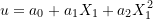Figure 6 shows the displacement function of a quadratic element with three nodes. An alternative form of the displacement is: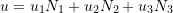where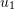,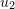, and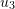are the displacements of the nodes 1, 2, and 3, while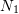, and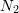, and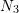are the shape functions associated with these nodes (Figure 6).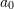,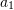, and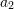are the generalized degrees of freedom of the element while,, andare the nodal degrees of freedom. To find the shape functions,, and, the following three equations are solved: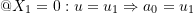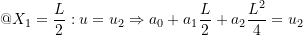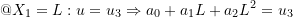Therefore,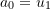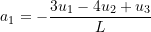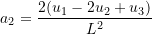Therefore, the displacement function in terms of the nodal degrees of freedom has the form: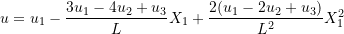Rearranging: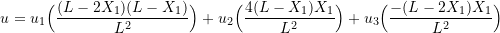Therefore, the shape functions are: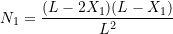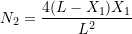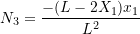It can be checked that the sum of the shape functions is always equal to 1, which allows for the rigid-body motion or constant displacement of an element to be modelled (Why?). The local stiffness matrix and the local nodal forces vectors can be established similar to the previous section. First, the following matrices are defined: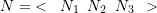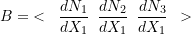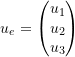then the displacement,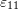, and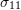can be written in terms of the nodal degrees of freedom vector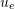as follows: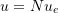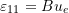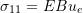If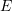is Young’s modulus and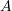is the cross sectional area of the element, then the local stiffness matrix of the element is a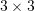matrix and has the form: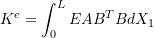Ifandare constant, then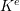has the form: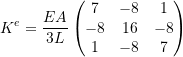The nodal forces vector is a 3 dimensional vector and has the form: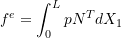where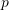is the distributed load per unit length acting on the element. Ifis constant, then the nodal forces vector have the form: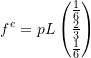View Mathematica Code

u = a0 + a1*X1 + a2*X1^2;
Eq1 = u /. X1 -> 0;
Eq2 = u /. X1 -> L/2;
Eq3 = u /. X1 -> L;
a = Solve[{Eq1 == u1, Eq2 == u2, Eq3 == u3}, {a0, a1, a2}]
u = u /. a[]
N1 = FullSimplify[Coefficient[u, u1]]
N2 = FullSimplify[Coefficient[u, u2]]
N3 = FullSimplify[Coefficient[u, u3]]
B = {{D[N1, X1], D[N2, X1], D[N3, X1]}};
B // MatrixForm
Transpose[B] // MatrixForm
K = EA*Integrate[Transpose[B].B, {X1, 0, L}];
K // MatrixForm
Nn = {{N1, N2, N3}};
fe = Integrate[p*Transpose[Nn], {X1, 0, L}];
fe // MatrixForm

View Python Code

import sympy as sp
from sympy import solve,Matrix,diff,integrate
a0,a1,a2,x,L,EA,p = sp.symbols("a_0 a_1 a_2 x L EA p")
u1,u2,u3 = sp.symbols("u_1 u_2 u_3")
u = a0+a1*x+a2*x**2
display("u =", u)
eq1 = u.subs({x:0})
eq2 = u.subs({x:L/2})
eq3 = u.subs({x:L})
s = solve((eq1-u1,eq2-u2,eq3-u3),a0,a1,a2)
display("Solve")
display("a_0 =",s[a0],"a_1 =",s[a1],"a_2 =",s[a2])
u = u.subs(s).expand()
display("Rearranging")
display("u =",u)
N1 = u.coeff(u1)
N2 = u.coeff(u2)
N3 = u.coeff(u3)
display("Shape Functions")
B = Matrix([[diff(N1,x),diff(N2,x),diff(N3,x)]])
display("B =",B.T)
K = EA*Matrix([[integrate(B[i]*B[j], (x,0,L)) for i in range(3)] for j in range(3)])
display("K^e =",K)
Nn = Matrix([[N1,N2,N3]]).T
display("N =",Nn)
fe = Matrix([p*integrate(Nn[i],(x,0,L))for i in range(3)])
display("Nodal Force Vector")
display("f^e =",fe)


### Problems

1. For a quadratic one dimensional element of length L, find the nodal forces vector if a distributed horizontal load of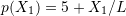(load per unit length) is applied on the element.

2. Using two quadratic one dimensional elements and the virtual work method, find the displacement of the nodes and the stress within each element of the following problem assuming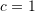,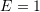,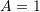, and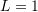units. Compare with the exact solution and the solution using four linear elements shown above.